Home | | Business Maths 12th Std | Testing the consistency of non homogeneous linear equations (two and three variables) by rank method

# Testing the consistency of non homogeneous linear equations (two and three variables) by rank method

Testing the consistency of non homogeneous linear equations (two and three variables) by rank method

Testing the consistency of non homogeneous linear equations (two and three variables) by rank method.

Consider the equations A X= B in ŌĆśnŌĆÖ unknowns.

(i) If Žü ([A, B] ) = Žü ( A) , then the equations are consistent.

(ii) If Žü[([A, B] ) = Žü ( A )= n , then the equations are consistent and have unique solution.

(iii) If Žü ([ A, B] ) = Žü ( A ) < n , then the equations are consistent and have infinitely many solutions.

(iv) If Žü ([A, B ]) ŌēĀ Žü ( A) then the equations are inconsistent and has no solution.

Example 1.9

Show that the equations x + y = 5, 2x + y = 8 are consistent and solve them.

Solution:

The matrix equation corresponding to the given system is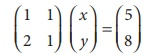AX=BNumber of non-zero rows is 2.

Žü (A )= Žü ([ A, B]) = 2 = Number of unknowns.

The given system is consistent and has unique solution.

Now, the given system is transformed into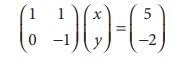x + y = 5

y = 2

Ōł┤ (1) ŌćÆ x + 2 = 5

x = 3

Solution is x = 3, y = 2

Example 1.10

Show that the equations 2x + y = 5, 4x + 2 y = 10 are consistent and solve them.

Solution:

The matrix equation corresponding to the system isŽü ( A ) = Žü ([ A, B]) = 1 < number of unknowns

Ōł┤ The given system is consistent and has infinitely many solutions.

Now, the given system is transformed into the matrix equation.Let us take y = k, k ŌłłR

ŌćÆ 2x + k = 5

x = 1/2 ( 5 ŌłÆ k)

x = 1/2 ( 5 ŌłÆ k) , y = k for all k ŌłłR

Thus by giving different values for k, we get different solution. Hence the system has infinite number of solutions.

Example 1.11

Show that the equations 3x ŌłÆ 2 y = 6, 6x ŌłÆ 4 y = 10 are inconsistent.

Solution:

The matrix equation corresponding to the given system is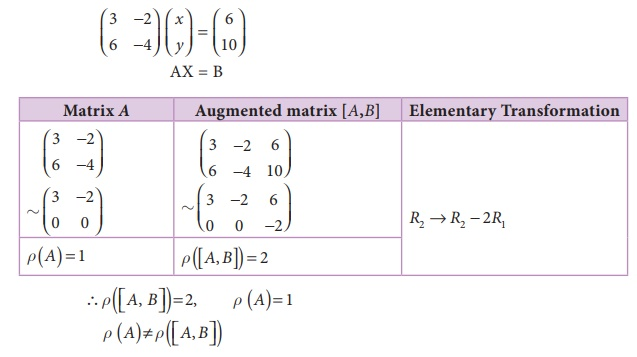Ōł┤The given system is inconsistent and has no solution.

Example 1.12

Show that the equations 2x + y + z = 5, x + y + z = 4, x ŌłÆ y + 2z = 1 are consistent and hence solve them.

Solution:

The matrix equation corresponding to the given system is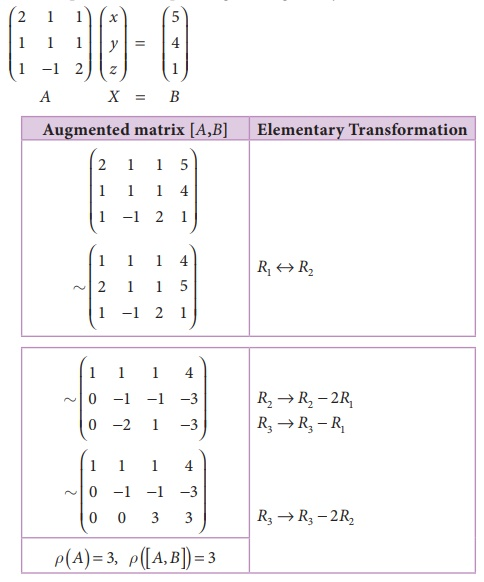Obviously the last equivalent matrix is in the echelon form. It has three non-zero rows.

Žü( A ) = Žü( [A, B] )= 3 = Number of unknowns .

The given system is consistent and has unique solution.

To find the solution, let us rewrite the above echelon form into the matrix form.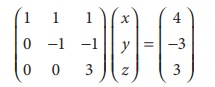x + y + z = 4 (1)

y + z = 3 (2)

3z = 3 (3)

(3)ŌćÆ z = 1

(2)ŌćÆ y = 3 ŌłÆ z = 2

(1) ŌćÆ x = 4 ŌłÆ y ŌłÆ z

x=1

Ōł┤ x = 1, y = 2, z = 1

Example 1.13

Show that the equations x + y + z = 6, x + 2 y + 3z = 14, x + 4 y + 7z = 30 are consistent and solve them.

Solution:

The matrix equation corresponding to the given system is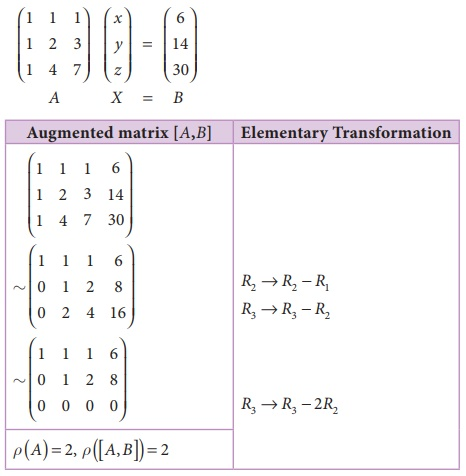Obviously the last equivalent matrix is in the echelon form. It has two non-zero rows.

Ōł┤ Žü ( [A, B] ) = 2, Žü ( A) = 2

Žü ( A ) = Žü ( [A, B] ) = 2 < Number of unknowns.

The given system is consistent and has infinitely many solutions.

The given system is equivalent to the matrix equation,x + y + z = 6  (1)

y + 2z = 8 (2)

(2)ŌćÆ y = 8 ŌłÆ 2z,

(1)ŌćÆ x = 6 ŌłÆ y ŌłÆ z = 6 ŌłÆ (8 ŌłÆ 2 z) ŌłÆ z = z ŌĆō 2

Let us take z = k, k ŌłłR , we get x = k ŌłÆ 2, y = 8 ŌłÆ 2k , Thus by giving different values for k we get different solutions. Hence the given system has infinitely many solutions.

Example 1.14

Show that the equations x ŌłÆ 4 y + 7z = 14, 3x + 8 y ŌłÆ 2z = 13, 7x ŌłÆ 8 y + 26z = 5 are inconsistent.

Solution:

The matrix equation corresponding to the given system isThe last equivalent matrix is in the echelon form. [A, B] has 3 non-zero rows and [A] has 2 non-zero rows.The system is inconsistent and has no solution.

Example 1.15

Find k, if the equations x + 2 y ŌłÆ 3z = ŌłÆ2, 3x ŌłÆ y ŌłÆ 2z = 1, 2x + 3y ŌłÆ 5z = k are consistent.

Solution:

The matrix equation corresponding to the given system isFor the equations to be consistent, Žü ( [A, B] ) = Žü ( A)= 2

Ōł┤21 + 7k = 0

7k = ŌłÆ21 .

k = ŌłÆ3

Example 1.16

Find k, if the equations x + y + z = 7, x + 2 y + 3z = 18, y + kz = 6 are inconsistent.

Solution:

The matrix equation corresponding to the given system isFor the equations to be inconsistent

Žü ( [A, B] ) ŌēĀ Žü ( A)

It is possible if k ŌłÆ 2 = 0 .

K=2

Example 1.17

Investigate for what values of ŌĆśaŌĆÖ and ŌĆśbŌĆÖ the following system of equations

x + y + z = 6, x + 2 y + 3z = 10, x + 2 y + az = b have

(i) no solution  (ii) a unique solution (iii) an infinite number of solutions.

Solution:

The matrix equation corresponding to the given system isCase (i) For no solution:

The system possesses no solution only when Žü ( A )ŌēĀ Žü ([ A, B]) which is possible only when a ŌłÆ 3 = 0 and b ŌłÆ 10 ŌēĀ 0

Hence for a = 3, b ŌēĀ 10 , the system possesses no solution.

Case (ii) For a unique solution:

The system possesses a unique solution only when Žü ( A ) = Žü ([ A, B]) = number of unknowns.

i.e when Žü ( A ) = Žü ([ A, B]) = 3

Which is possible only when a ŌłÆ 3 ŌēĀ 0 and b may be any real number as we can observe .

Hence for a ŌēĀ 3 and b ŌłłR , the system possesses a unique solution.

Case (iii) For an infinite number of solutions:

The system possesses an infinite number of solutions only when

Žü ( A )= Žü ([ A, B]) < number of unknowns

i,e when Žü ( A)= Žü ([ A, B])= 2 < 3 ( number of unknowns) which is possible only when a ŌłÆ 3 = 0, b ŌłÆ 10 = 0

Hence for a = 3, b =10, the system possesses infinite number of solutions.

Example 1.18

The total number of units produced (P) is a linear function of amount of over times in labour (in hours) (l), amount of additional machine time (m) and fixed finishing time (a)

i.e, P = a + bl + cm

From the data given below, find the values of constants a, b and cEstimate the production when overtime in labour is 50 hrs and additional machine time is 15 hrs.

Solution:

We have, P = a + bl + cm

Putting above values we have

6,950 = a + 40b + 10c

6,725 = a + 35b + 9c

7,100 = a + 40b + 12c

The Matrix equation corresponding to the given system isŌł┤ The given system is equivalent to the matrix equationŌł┤ The production equation is P = 5000 + 30l + 75m

Pat l = 50, m=15         = 5000 + 30(50) + 75(15)

=7625 units.

Ōł┤The production = 7,625 units.

Tags : with Solved Example Problems , 12th Business Maths and Statistics : Chapter 1 : Applications of Matrices and Determinants
Study Material, Lecturing Notes, Assignment, Reference, Wiki description explanation, brief detail
12th Business Maths and Statistics : Chapter 1 : Applications of Matrices and Determinants : Testing the consistency of non homogeneous linear equations (two and three variables) by rank method | with Solved Example Problems